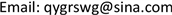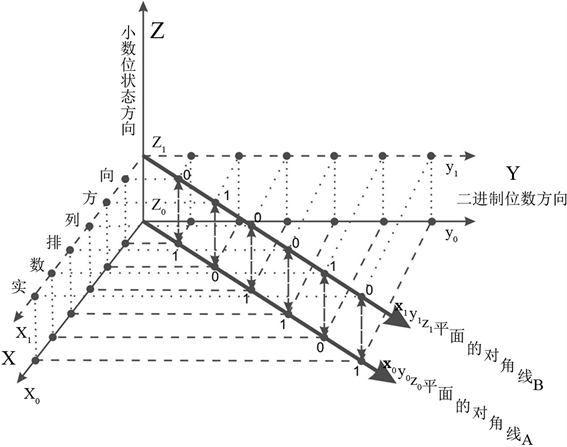﻿ 数学基础若干问题的创新性思考 Innovative Thinking on Several Basic Problems of Mathematics

Pure Mathematics
Vol. 08  No. 05 ( 2018 ), Article ID: 26758 , 18 pages
10.12677/PM.2018.85069

Innovative Thinking on Several Basic Problems of Mathematics

Weiguo Shen

Former Institute of Logic and Artificial Intelligence, Northwest University of Technology, Xi’an ShaanxiReceived: Aug. 20th, 2018; accepted: Sep. 4th, 2018; published: Sep. 11th, 2018ABSTRACT

On the basis of previous series of papers, cantor diagonal method is put forward and the nested interval method proves that the obvious logic problems in the process of real number are uncountable. Its essence is that the use of reduction to absurdity in this specific case in the past is difficult to detect. Although the uncountability of the set of real numbers is widely accepted, it causes many problems. It makes the so-called mathematical basis extremely complex and full of contradictions. Even more complex and confusing than the other branches of mathematics that are expected to provide a solid foundation, this only suggests that the theory itself has problems. There are two aspects to the practical significance of this paper. One is the mathematical basis and set theory. Second, in the aspect of logic, people should be reminded of the mistakes in the use of proof by contradiction, and that people should adopt a more rigorous and prudent attitude towards any logical, mathematical conclusion and proof. In this paper, we also discuss the problems related to Godel’s theorem and the simplest theoretical problems about the concept of non-limit and infinitesimal of differential method.

Keywords:Cantor Diagonal Method, Interval Sleeve Method, Real Number, Numbered, Do Not Count, Reduction to Absurdity, Quasi-Binary Representation of Real Numbers, Godel’s Theorem, Turing Machine, Artificial Intelligence, Differential and Integral Calculus1. 康托对角线法中的逻辑推理问题详析

2. 戴德金分割中的矛盾分析

3. 康托超限数理论中的隐含矛盾

4. 康托定理与康托对角线法的同构性分析

$0.10\cdots =f\left(x\right)\ne x=01$ ，这里左边列出的是康托二维表中的第二个实数，其小数点后的第二位是0；而最右边的x = 01，是指对角线上对第二个实数的第二位的“0”求反后的那个数“1”，加上前面的第一步得到的“0”，为“01”；

$0.001\cdots =f\left(x\right)\ne x=010$ ，这里左边列出的是康托二维表中的第三个实数，其小数点后的第三位是1；而最右边的x = 010，是指对角线上对第三个实数的第三位的“1”求反后的那个数“0”，加上前面的第一、二步得到的“01”，为“010”；

5. 再论“层叠集合观”的困境及与康托定理有关的内在矛盾及测度论所隐含的问题

6. 可数、不可数概念的概率角度分析

7. 人工智能的新领域：计算机在康托对角线法的反证法证明无效性的验证上的应用可能

8. 对比、印证与启发：将可数的有理数运用于康托对角线法可能出现的情况

9. 总结：康托对角线法推导不成立、实数集可数，是数学基础的返璞归真

10. 人工智能、算法与人脑、人的智力孰强问题的由来及哥德尔定理及其相关问题详析

11. 图灵机停机问题的讨论Figure 1. Diagram of binary double diagonal lines

12. 一种不需要极限及无穷小概念的微积分新诠释

1、导数定义(第一定义)：曲线上某点的切线斜率。

2、牛顿、莱布尼兹求导法的实质

$\Delta h/\Delta g\left(当\Delta x=0,\Delta g=1\text{ }时\right)=K\left(x,\Delta x\right)\cdot \Delta g/\Delta g=K\left(x,0\right)\cdot 1/1=K\left(x,0\right)$ (1)

$K\left(x,\Delta x\right)=K\left({x}_{1},0\right)$ (2)

$K\left({x}_{1},0\right)\cdot \Delta x=K\left(x,\Delta x\right)\cdot \Delta x=\Delta y$ (3)

$2x+\Delta x=2{x}_{1}$ (4)

(4)式等号左边为割线斜率(第二定义下的导数)，右边为x1点的切线斜率(第一定义下的导数)，由 ${x}_{1}=x+\Delta x/2$ 可知， $x<{x}_{1} ,x1即为中值。如将4式两边乘以自变量Δx，则可得函数微分，即

$2{x}_{1}\cdot \Delta x=\left(2x+\Delta x\right)\cdot \Delta x=2x\cdot \Delta x+\Delta {x}^{2}=\Delta y$ (5)

${\int }_{a}^{b}f\left(x\right)\text{d}x=F\left(b\right)-F\left(a\right)=f\left(x\right)\left(b-a\right)=f\left(x\right)\Delta x$ (6)

Innovative Thinking on Several Basic Problems of Mathematics[J]. 理论数学, 2018, 08(05): 516-533. https://doi.org/10.12677/PM.2018.85069

1. 1. 沈卫国. 论数学基础问题, 论自然科学的若干基本问题[M]. 福州: 海风出版社, 1998.

2. 2. 沈卫国. 论熵、不可逆过程及数学中的无穷[M]. 福州: 海风出版社, 2009.

3. 3. 沈卫国. 论康托对角线法的局限性与数学、逻辑学中的一些基础性问题[J]. 天津职业院校联合学报, 2008(3).

4. 4. 沈卫国. 论序数及连续统的可数性问题与正则公理[J]. 天津职业院校联合学报, 2011(5).

5. 5. 沈卫国. 关于康托对角线法推导问题的进一步解释及说明[M]. 国家科技图书文献中心预印本.

6. 6. 沈卫国. 再论康托对角线法中隐含的简单形式逻辑问题[M]. 国家科技图书文献中心预印本.

7. 7. 沈卫国. 康托对角线法及其错误的实质[M]. 国家科技图书文献中心预印本.

8. 8. 沈卫国. 康托对角线法推导中的逻辑误区及其函数关系的再分析[M]. 国家科技图书文献中心预印本.

9. 9. 沈卫国. 康托对角线法问题详析及由此引出的反证法使用中必须注意的误区[C]. 第11次全国科学逻辑研讨会论文集: 2016年卷. 芜湖: 安徽师范大学, 2016.

10. 10. 沈卫国. 康托对角线法中的逻辑问题及由此引出的反证法使用中必须注意的推理误区[J]. 前沿科学, 2017(2).

11. 11. 沈卫国. 增量分析视野下的测度问题、微积分求导及连续统的可数性[J]. 前沿科学, 2017(3).

f：X→2X。现考虑集合

A：={xϵX：xɇf(x)}